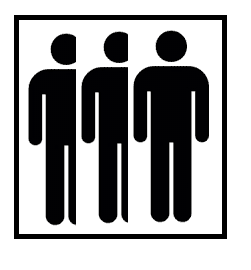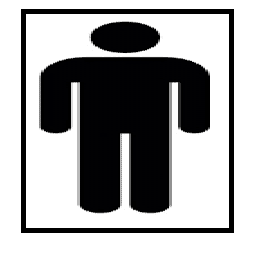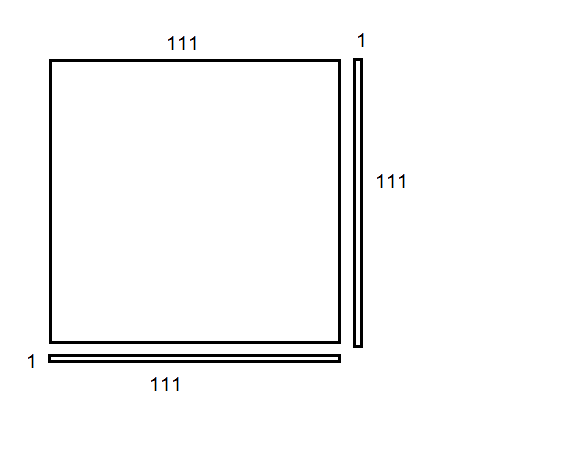# What exactly is this concept called? -- Finding "?" in x^2+16x+? to get (x+8)^2

x^2+16x+?
That ? Can only be 64 so the polynomial can transform into (x+8)^2 where the position where in this case “8” is must be an integer.

What exactly are these kind of concepts called?

fresh_42
Mentor
2021 Award
We call it "quadratic supplement" and Wiki translates it to "completing the square".

•jedishrfu
jedishrfu
Mentor
We call it "quadratic supplement" and Wiki translates it to "completing the square".

Sounds like something you take when you need more vitamins vs being a carpenter and building a square.

•pbuk
fresh_42
Mentor
2021 Award
Sounds like something you take when you need more vitamins vs being a carpenter and building a square.
Well, completing the square sounds like a paver at work.

•solarmidnightrose and jedishrfu
berkeman
Mentor
LOLfresh_42
Mentor
2021 Award
Sounds like something you take when you need more vitamins
Nutritional supplements aren't famous enough here - outside sports - that this association would come up. It would rather beor#### Attachments

•YoungPhysicist and jedishrfu
I don’t know if this is right,but after I searched “quadratic supplement”online,it seems like the process of turning the form of
ax^2+bx+c
to
a(x-h)^2+k

but in my case it kind of means the process of filling in a specific coefficient (either a or b or c)
in a way that the entire polynomial can be turn into (x+n)^2 where n must be an real number(sorry for the mispost up there)and the transformation process is based on
(a+b)^2 = a^2 +2ab+ b^2

Ps:It is like the specific propertie of a set of quadratic polynomials that its a(x-h)^2+k form has a = 1 and k = 0

fresh_42
Mentor
2021 Award
The general idea is always to transform a quadratic polynomial ##a_0x^2+a_1x+a_2## into a form ##c\cdot (x \pm a)^2 +b## in order to isolate ##(x \pm a)^2## and solve it.

•FactChecker and YoungPhysicist
Get it.Thanks!!

•berkeman
Mark44
Mentor
Well, completing the square sounds like a paver at work.
In the US (and possibly UK?), we call this technique "completing the square."

•symbolipoint
fresh_42
Mentor
2021 Award
In the US (and possibly UK?), we call this technique "completing the square."
Yes, that's what Wikipedia (my preferred dictionary for technical terms) gave me. My own translation "quadratic supplement" is not really optimal for "quadratische Ergänzung", which could also be translated by "quadratic completion" which is pretty close. I found the English name a bit funny, as square has multiple meanings in English (geometric figure, "don't be square", Times or Trafalgar square), whereas quadratic has not.

Mark44
Mentor
"Quadratic supplement" is not that far off from "completing the square," since the Latin for "square" is quadratus.
There are also a few other meanings for "square," such as "a square deal" (fair deal), or "to square accounts," (pay off a debt).

•fresh_42
pbuk
Gold Member
Yes it is "completing the square" in the UK. I have always assumed that the term comes from the geometric construction shown in the animation on the Wikipedia page: solving the problem involves finding the "missing piece" that literally completes the construction of a square. I don't normally use Wikipedia for mathematical terms, preferring Mathworld.

fresh_42
Mentor
2021 Award
Yes it is "completing the square" in the UK. I have always assumed that the term comes from the geometric construction shown in the animation on the Wikipedia page: solving the problem involves finding the "missing piece" that literally completes the construction of a square. I don't normally use Wikipedia for mathematical terms, preferring Mathworld.
I spoke about translations. Wikipedia allows to switch from my native language directly to the corresponding English term, WolframAlpha does not, neither do ordinary dictionaries. It has also the advantage, that those pages do not correspond one on one, so you can sometimes find better descriptions, sources, formulas or graphics on other language sites. And for all who are really interested in accurate definitions and links, I strongly recommend nLab rather than WolframAlpha.

I would love to know , as I have asked before, why the "missing piece" in #14 cannot be given a name. adquadratus ?
For
odd integers ,e.g. 223, -
##1112 +223 =1122##
would it then be alright to say 223 is the adquadratus ??

also in #13 don't forget the square meal ! [ from the wooden square plates on RN vessels of old , kept in a slotted rack so they they did not roll out in heaving sea states]

Last edited:
FactChecker
Gold Member
As a "fun" exercise, you can use the technique of completing the square to derive the quadratic formula.

•Janosh89, fresh_42 and YoungPhysicist
Mark44
Mentor
I would love to know , as I have asked before, why the "missing piece" in #14 cannot be given a name. adquadratus ?
Janosh89 said:
For odd integers ,e.g. 223, -
##1112 +223 =1122##
would it then be alright to say 223 is the adquadratus ??
I think you would be alone in the world to call it that. The "ad" in ad quadratum doesn't mean "to add" -- the Latin preposition means "toward" or "to".

Regarding your numbers, ##111^2 + 223 = 111^2 + 222 + 1 = 111^2 + 2 \cdot 111 + 1 = (111 + 1)^2 = 112^2##. Geometrically, the two terms , ##111^2## and ##222##, could be visualized as a square whose sides are 111 and two rectangles, each of length 111 and width 1. Completing the square means determining which square would fill in the small gap at the lower right in my drawing. In this case, a 1 X 1 square would do the job.The areas of the 111 X 111 square, the two thin rectangles, and the small 1 X 1 square (not shown) add up to the combined figure, a 112 X 112 square.
Janosh89 said:
also in #13 don't forget the square meal ! [ from the wooden square plates on RN vessels of old , kept in a slotted rack so they they did not roll out in heaving sea states]

#### Attachments

•Janosh89 and pbuk
fresh_42
Mentor
2021 Award
As a "fun" exercise, you can use the technique of completing the square to derive the quadratic formula.
Fun??? That's often the only way for me to check if I remembered the signs correctly.•Janosh89 and FactChecker
FactChecker
Gold Member
Fun??? That's often the only way for me to check if I remembered the signs correctly.Ha! IMHO, one of the signs of a mathematician is the reliance on quick derivations rather than memorization (although, I understand that Gauss had a photographic memory -- just another thing that made him the ultimate genius.)

Last edited:
•Janosh89 and fresh_42
fresh_42
Mentor
2021 Award
Ha! IMHO, one of the signs of a mathematician is the reliance on quick derivations rather than memorization (although, I understand that Gauss had a photographic memory -- just another way that he was the ultimate genius.)
This would explain why my library is far better than my memory <sigh>.

•FactChecker
Found out / discovered that addendum is the gerund of the Latin verb addere . [to add together]
Painful memories of chalk dust flooding back. ..

Last edited: